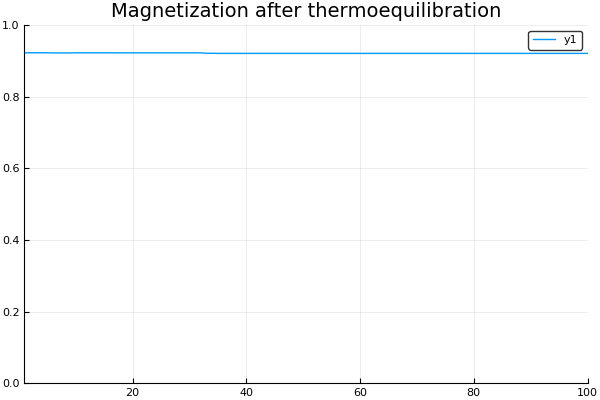3

1

1

2

# Social Systems - Statistical Mechanics

Simple modelling of some types of social systems using Statistical Mechanis and MCMC Simulations.

Install the package running:

``````julia> Pkg.clone("https://github.com/kaslusimoes/SocialSystems.jl.git")
``````

## A simple example

We define a BasicSociety and perform MCMC until equilibration around an issue. After that, we can verify the magnetization of the society is stabilized.

``````julia> using SocialSystems, Plots

julia> N   = 100
julia> ρ   = 0.2
julia> ε   = 0.1

julia> soc     = BasicSociety(n, ρ, ε)
julia> iter, x = metropolis!(soc, β=15.)

julia> nsteps = 100
julia> mi = zeros(nsteps)
julia> for i in 1:nsteps
metropolisStep!(soc, x, 15.);
mi[i] = magnetization(soc, x)
end

julia> plot(1:nsteps, mi, title = "Magnetization after thermoequilibration")
``````09/23/2015

9 days ago

72 commits